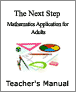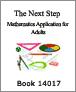# Arithmetic operations

## Operations with Mixed Fractions Part 2- Practice [Video – 9:04] (2011)This math video tutorial offers learners a chance to practise multiplication and division with mixed fractions. A mixed fraction is one that contains both a whole number and a fraction.

The tutorial includes three math problems involving mixed fractions. Learners are asked to pause the video while they work out the problems on their own, then restart it to see the instructor’s solutions.

2012-05-03

## Operations with Mixed Fractions Part 2 – Concept [Video – 9:04] (2011)This math video tutorial introduces multiplication and division with mixed fractions.

The instructor explains the steps required, including converting a mixed fraction to an improper one; carrying out the operation; and reducing the resulting fraction to its simplest form.

He points out that with multiplication and division problems, unlike addition and subtraction ones, there is no need to find a common denominator.

2012-05-03

## Operations with Mixed Fractions Part 3 - Practice [Video – 11:05] (2011)This video tutorial offers further practice in carrying out math problems involving mixed fractions.

It presents three problems of increasing complexity. Learners are asked to pause the video, work out the problems, then restart the video to see the instructor’s detailed solutions.

2012-04-11

## Operations with Mixed Fractions Part 3 – Concept [Video – 12:09] (2011)This math video tutorial looks at order of operations as it is applied to problems containing mixed fractions. The order of operations specifies that if a problem contains some combination of calculations, the multiplication and division components must be completed before the addition and subtraction ones.

2012-04-11

## Operations with Mixed Fractions Part 1- Practice [Video – 7:22] (2011)This math video tutorial offers further practice in adding and subtracting with mixed fractions.

It presents three problems of increasing complexity. Learners are asked to pause the video, work out the problems, then restart the video to see the instructor’s detailed solutions.

2012-04-03

## Operations with Mixed Fractions Part 1 – Concept [Video – 13:17] (2011)In this math video tutorial, learners begin to carry out arithmetic operations involving mixed fractions.

The instructor explains the steps needed for addition or subtraction problems, including converting a mixed fraction to an improper fraction; finding a common denominator; and reducing the fraction.

He uses three problems as examples, breaking the solutions down into small steps.

2012-04-03

## The Next Step: Teacher's Manual (2003)The Teachers Manual section of the "Next Step" Mathematics Applications for Adults provides the instructor with course material necessary for Books 14011 - 14019 such as Answer Keys; Post-Tests; Post-Test Answer Keys; Multiple-Choice Test Strategies; Customary Measurement; Formulas.

2003-08-08

## The Next Step: Mathematics Applications for Adults - Book 14018 (2003)The Book 14018 section of the "Next Step" manual for adults demonstrates the mathematics applications for Number Operations; Fractions, Decimals, and Percent; Percent, Ratio, and Proportion; Geometry; Measurement, Integers; Equations: Equalities and Inequalities; Graphs.

2003-08-08

## The Next Step: Mathematics Applications for Adults - Book 14017 (2003)The Book 14017 section of the "Next Step" manual for adults demonstrates the mathematics applications for Number Operations; Fractions, Decimals, and Percent; Percent and Ratio; Measurement, Integers; Equations: Equalities and Inequalities; Graphs.

2003-08-08

## The Next Step: Mathematics Applications for Adults - Book 14016 (2003)The Book 14016 section of the "Next Step" manual for adults demonstrates mathematics applications for Whole Numbers (number/word recognition, place value, counting, addition, subtraction, multiplication, division, word problems), Fractions (understanding and comparing, addition, subtraction, multiplication and division of fractions, word problems with fractions), Decimals (understanding and comparing, addition, subtraction, multiplication and